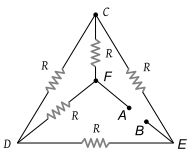NEET Physics Current Electricity Questions Solved

Five equal resistances each of resistance R are connected as shown in the figure. A battery of V volts is connected between A and B. The current flowing in AFCEB will be(1) $\frac{3V}{R}$

(2) $\frac{V}{R}$

(3) $\frac{V}{2R}$

(4) $\frac{2V}{R}$

Explanation is a part of a Paid Course. To view Explanation Please buy the course.

Difficulty Level: